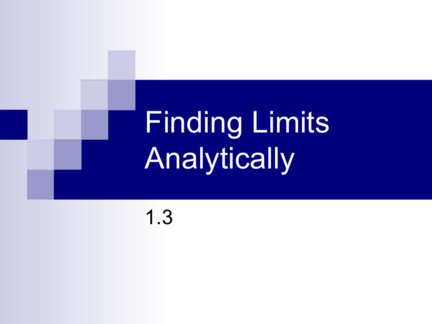# Finding Limits AnalyticallyContributed by:CONCEPTS COVERED:
1. Properties of Limits
2. Strategies for finding limits
3. The Squeeze Theorem
1. Finding Limits
2. Concepts Covered:
 Properties of Limits
 Strategies for finding limits
 The Squeeze Theorem
3.  From section 1.2: The limit of f(x) as x
approaches c does not depend on the
value of f at x = c.
 Example:
 However, sometimes the limit may be
exactly f(c). When this occurs the function
is at x = c. In this case find
the limit by substitution.
 Example:
4. Basic Limits:
1. lim b b lim x c lim
n n
2. 3. x c
x c x c x c
3
lim12 
x 4
lim x 
x 4
lim x
x 4
5. Properties of Limits:
lim f ( x) L lim g ( x) K
x c
x c
1. Scalar Multiple:
2. Sum or Difference:
3. Product:
4. Quotient:
5. Power:
6. Example using limit properties:
lim
3 2
( 2 x  4 x  1) 
x  2
7. Example using limit properties:
1
lim
x c
f ( x) 4 lim
x c
g ( x) 
2
Find the following:
lim[3 f ( x)] 
x c
lim[ f ( x)  g ( x)] 
x c
lim
1/ 2
lim[ f ( x) g ( x)]  [ f ( x )] 
x c x c
8. Example using limit properties:
2
x  2x  3
lim 
x 2 x2
x2  2x  3
lim 
x  2 x2
9. Limits of Trigonometric Functions:
You can evaluate the limits of trig functions using
direct substitution if
.
lim (cos x) 
x  / 2
lim (sin x) 
x  / 2
lim (sec x) 
x  / 2
10. Did you understand?
1. When can you use direct substitution to
find a limit?
11. If direct substitution will not work….
Try one of these techniques:
1. Cancellation
2. Rationalization
12. Try to find a function that agrees with your
function at all but .
2
x 4
lim 
x 2 x  2
13. Rationalize the numerator.
x 1  1
lim
x 0 x
14. The Squeeze Theorem:
 If h(x) ≤ f(x) ≤ g(x) for all x in an open
interval containing c, except for possibly at
c itself and if lim h( x) L lim g ( x) , then
x c x c
 Example: If 4 – x2 ≤ f(x) ≤ 4 + x2, find
lim f ( x)
x 0
15. Two Special Trig Limits:
sin x 1  cos x
1. lim 1 lim
2. 0
x 0 x x 0 x
Examples:
tan x
lim 
x 0 x
cos  tan 
lim 
0 
16. Steps to follow for finding Limits: